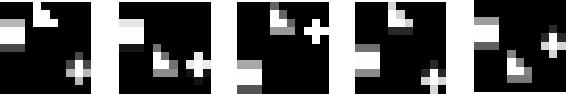## Multiple Cause Vector Quantization

### Performance Experiments:

The following experiments were conducted to study the performance of MCVQ as the number of vector quantizers (VQ's) and number of vectors per VQ are varied.

### Data Sets:

The experiments were carrired out on two data sets of gray-scale images, the first depicting geometric shapes and the second depicting faces.

#### a) Shapes

The shapes data set consists of 11-by-11 pixel images, as depicted below. Each image contains three shapes, with fixed horizontal position, but variable vertical position. Since each shape is roughly 3-by-3 pixels, there are 9^3=729 different shape images possible, if each shape must lie on a pixel boundary. These images were enumerated, then each shape was `jittered' vertically by a random value, uniformly distributed between -1 and +1 pixels. Anti-aliasing was employed to render shapes not lying exactly on pixel boundaries.The training set consisted of 100 of these images, randomly selected. The remaining 629 images comprised the testing set.

#### b) Faces

The faces data employed was the CBCL FACE DATABASE #1, made available by the MIT Center For Biological and Computation Learning. It consists of 19-by-19 pixel images, each containing a single frontal or near-frontal face. A training set of 1800 images, and a testing set of 629 images was chosen. (This was the same split used for the Multiple Cause Vector Quantization paper.)

### Procedure:

#### a) Shapes

Performance at reconstructing the training images was evaluated with the number of VQ's, K, ranging from 1 to 12, and the number of vectors per VQ, J, also ranging from 1 to 12.

For each pair (J,K), an MCVQ model was trained. Convergence of EM was assumed when the free energy had not changed by more than 1e-3 % during the previous three iterations.

For each testing image, the expected values of the latent variables (m's) were inferred. These values were used, with the learned model, to generate a reconstruction of the image. The root-mean-squared error between the reconstruction and the original was calculated, and summed over all the training images.

To smooth out the effects of converging to a local minimum, the above experiment was repeated 10 times for each (J,K).

Annealing was employed for the learning of the m's and the g's, using the schedule: {3, 2.7, 2.4, 2.1, 1.8, 1.5, 1.2, 1, 1, 1, ...}.

#### b) Faces

As above, with the following differences:

• K and J ranged from 1 to 8.
• Convergence was decided using a free energy tolerance of 1e-2 %.
• The experiment was repeated only 5 times.